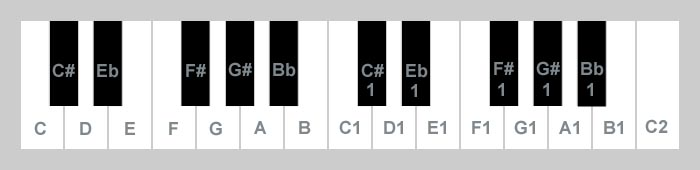First Music Lesson
Frequency, Octave, Semitone, Names of Sounds, Major Scale
Next Lesson >>

Frequency

We can describe sounds in terms of frequency. You can click on the buttons below to hear the sounds of piano.

When you hear the first sound the membrane in your speaker makes 440 vibrations per second, and when you hear the second sound it makes 220 vibrations per second. The frequency is measured in Hz. (1Hz = one vibration per second)

Octave and Semitone

We can measure the distance between the frequencies of two sounds by deviding the frequency of one by the frequency of the other. Such a distance is called interval. An octave is the interval between two sounds one of which has twice the frequency of the other. We devide octave into 12 semitones. In our terms, one semitone = 1.05946... (basically the 12th root of 2).

Names of sounds
Apronus convention

The sound with frequency 440Hz is called here A1. The sound which is an octave lower is called A and has the frequency of 220Hz. We define the other names below.

 130.8 Hz 261.6 Hz 138.6 Hz 277.2 Hz 146.8 Hz 293.7 Hz 155.6 Hz 311.1 Hz 164.8 Hz 329.6 Hz 174.6 Hz 349.2 Hz 185.0 Hz 370.0 Hz 195.0 Hz 392.0 Hz 207.7 Hz 415.3 Hz 220.0 Hz 440.0 Hz 233.1 Hz 466.2 Hz 246.9 Hz 493.9 Hz

Note that 130.8Hz x 1.05946 = 138.6Hz which means that the interval between C and C# is one semitone. It is also one semitone between C# and D, D and Eb, Eb and E, and so on. The frequency of C1 is 261.6Hz. Note that 130.8Hz x (1.05946)^12 = 130.8Hz x 2 = 261.6Hz. The interval between C and C1 is one octave and the interval between B and C1 is one semitone. You can continue the above table. For example you can have lower C=65.4Hz and C2=523.2Hz and so on and so forth.

Note that in our Apronus didactic notation A(-1) = 110Hz, A = 220Hz, A1 = 440Hz, A2 = 880Hz, etc. You must have in mind that there are some other conventions. For example in so called scientific notation A0 = 55Hz, A1 = 110Hz, A3 = 220Hz, A4 = 440Hz, etc. There is also Helmholtz system where A = 110Hz, a = 220Hz, a' = 440Hz, a'' = 880Hz.

Scale

When you click on the button below you will hear the diatonic scale C major.

You can play it manually by pressing the buttons below from left to right.

Note that in the C major scale there are only "pure" capitals without # and b.

Now look at the C major scale in terms of semitones.

 sounds interval from Cin semitones 0 2 4 5 7 9 11 12

You can start a major scale with another sound. Let us start with C#.

 sounds interval from C#in semitones 0 2 4 5 7 9 11 12

And let us start again with F.

 sounds interval from Fin semitones 0 2 4 5 7 9 11 12

Note that each scale above is different from the others, but they sound very alike. They start with different sounds but each of them has the same intervals. Intervals are crucial in the process of song recognition.

Now you can perceive how sounds are located on piano keyboard.Note that the C major scale is the only major scale that you can play using only white keys.

You can use our virtual piano to experiment with the musical knowledge that you have gained in this lesson.